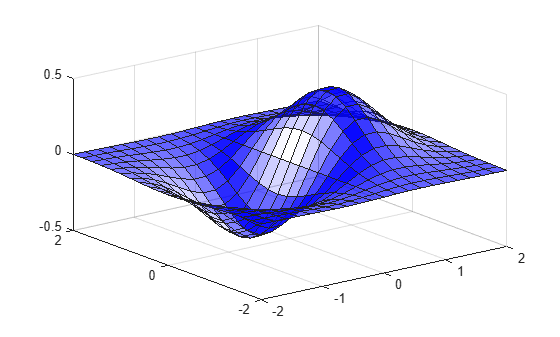# alphamap

Specify figure alphamap (transparency)

## Syntax

```alphamap(alpha_map) alphamap('parameter') alphamap('parameter',length) alphamap('parameter',delta) alphamap(fig,...) alphamap(ax,...) alpha_map = alphamap alpha_map = alphamap(fig) alpha_map = alphamap(ax) alpha_map = alphamap('parameter') ```

## Description

`alphamap(alpha_map)` sets the `AlphaMap` of the current figure to the specified `m`-by-1 array of alpha values, `alpha_map`. If you set the alphamap for the figure, then axes and charts in the figure use the same alphamap.

`alphamap('parameter')` creates a new alphamap or modifies the current alphamap. You can specify the following parameters:

• `'default'` — Set the `AlphaMap` property to the figure's default alphamap.

• `'rampup'` — Create a linear alphamap with increasing opacity (default `length` equals the current alphamap length).

• `'rampdown'` — Create a linear alphamap with decreasing opacity (default `length` equals the current alphamap length).

• `'vup'` — Create an alphamap that is opaque in the center and becomes more transparent linearly towards the beginning and end (default `length` equals the current alphamap length).

• `'vdown'` — Create an alphamap that is transparent in the center and becomes more opaque linearly towards the beginning and end (default `length` equals the current alphamap length).

• `'increase'` — Modify the alphamap making it more opaque (default `delta` is `.1`, added to the current values).

• `'decrease'` — Modify the alphamap making it more transparent (default `delta` is `.1`, subtracted from the current values).

• `'spin'` — Rotate the current alphamap (default `delta` is 1; `delta` must be an integer).

`alphamap('parameter',length)` creates a new alphamap with the length specified by the integer `length` (used with parameters `'rampup'`, `'rampdown'`, `'vup'`, `'vdown'`).

`alphamap('parameter',delta)` modifies the existing alphamap using the value specified by the integer `delta` (used with parameters `'increase'`, `'decrease'`, `'spin'`).

`alphamap(fig,...)` performs the operation on the alphamap of the figure identified by `fig`.

`alphamap(ax,...)` performs the operation on the alphamap of the `Axes` object, `PolarAxes` object, or `GeographicAxes` object identified by `ax`.

`alpha_map = alphamap` returns the current alphamap.

`alpha_map = alphamap(fig)` returns the current alphamap from the figure identified by `fig`.

`alpha_map = alphamap(ax)` returns the current alphamap from the `Axes` object, `PolarAxes` object, or `GeographicAxes` object identified by `ax`.

`alpha_map = alphamap('parameter')` returns the alphamap modified by the `parameter`, but does not set the `AlphaMap` property.

## Examples

collapse all

Create a surface plot and change the alphamap.

```[x,y] = meshgrid([-2:.2:2]); z = x.*exp(-x.^2-y.^2);```

Plot the data, using the gradient of `z` as the alphamap.

```figure surf(x,y,z+.001,'FaceAlpha','flat',... 'AlphaDataMapping','scaled',... 'AlphaData',gradient(z),... 'FaceColor','blue')```Change the alphamap to be opaque at the middle and transparent towards the ends.

`alphamap('vup')`Starting in R2018a, if you set the alphamap for a figure, then axes and charts in the figure use the same alphamap. Previously, any axes or chart that you set the alphamap for explicitly were unaffected when you set the figure alphamap. If you want an `Axes` object to use a different alphamap than the figure, then set the axes alphamap after setting the figure alphamap.